# How to Use LEGO Bricks to Teach Hands-on Math

LEGO bricks make math practice a lot more fun!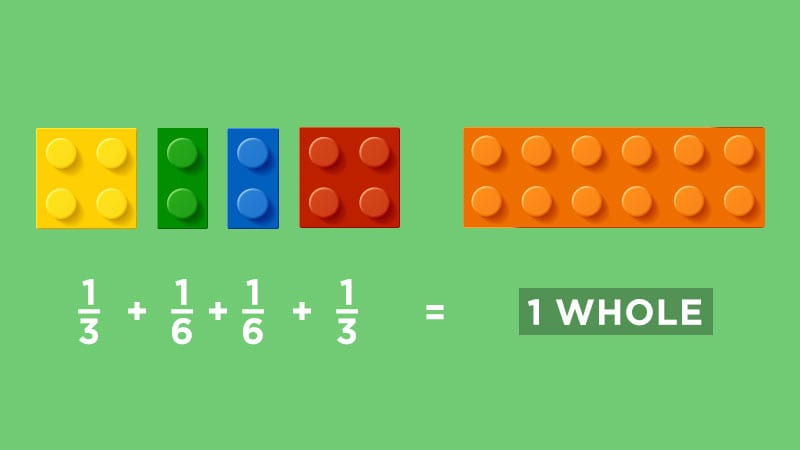We know students love playing with LEGO bricks, so they make a genius math manipulative for the classroom. Here are several ways to bring LEGO math into your classroom.

## Promote fraction comprehension.

LEGO bricks are such a fun and easy way to teach fractions in your class. Just bring in a small bag, and you’ll have enough for the entire class to work with and follow along at their desk as you teach fraction concepts to the group. The above video shows you how we did it. If you’re looking to do even more, try a fractions game. Here’s a LEGO roll game printable you can save and print.

## Put area and perimeter into practice.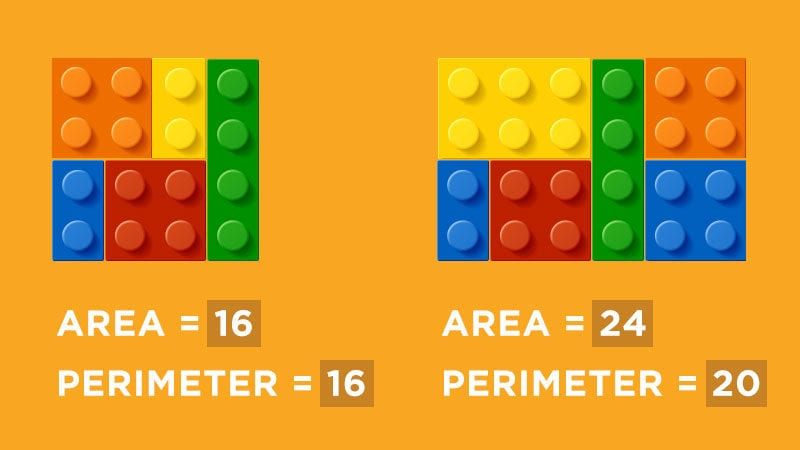Students can create big areas by putting LEGO bricks side by side or find the area of single bricks by simply counting the studs on top of the bricks and solving the equation. Students can also find the area and perimeter using grid paper. Tell students not to stress if bricks don’t fit perfectly on the grid paper. Have them color the area of each of their LEGO bricks on their grid paper.

## Use LEGO bricks for understanding multiplication.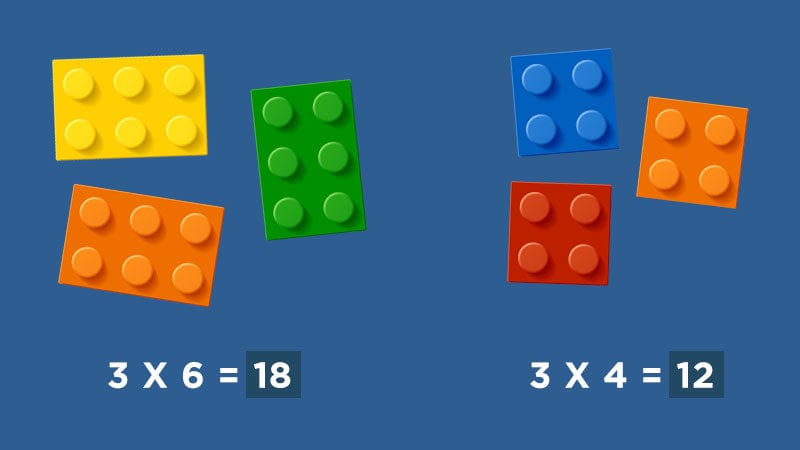If you’re trying to teach multiplication groups, a single LEGO can represent one group. Then students should count the studs to figure out the multiplication equation. For example, two LEGO bricks with four studs (two groups of four) is 2 x 4. Once students have figured that out, they can solve the problem (2 x 4 = 8).

For teaching array, kids should examine the rows to figure out the equation. For example, one LEGO brick with 8 studs (2 horizontal rows of 4 studs, 2 x 4 = 8).

## Teach lessons of mean, median, and range.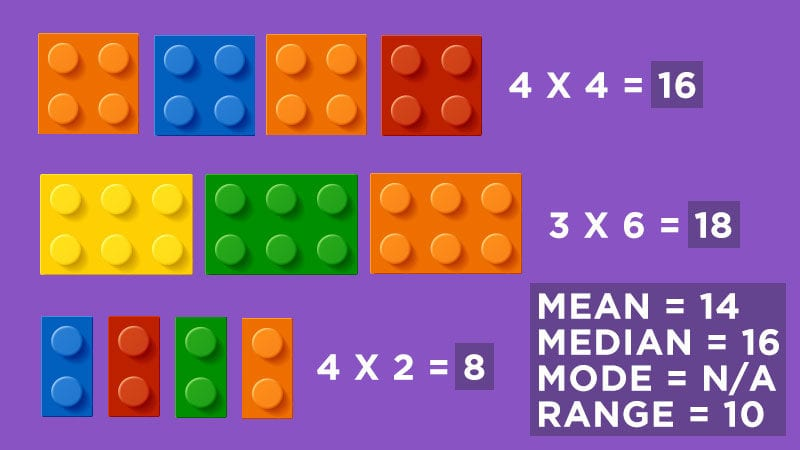Hand each student a baggie of LEGO bricks of various sizes and explore mean, median, mode, and range!

For array studs:
1. Students classify bricks by the number of studs.
2. Then, have them figure out the total number of studs for each group.
3. Once they have their numbers, they can figure out the m, m, m, and r.

For the number of bricks with the same number of studs:
1. Students classify bricks by number of studs.
2. Then, they count how many they have in each group.
3. Then, they figure out the m, m, m, and r.

You could also build LEGO towers and classify bricks by color. Then, have students figure out the mean, median, mode, and range. Set a timer and have kids build the tallest tower they can before the time runs out! Then, they take their tower apart and classify their bricks by color. Using their data (ex: 19 red, 10 blue, etc.), they figure out the m, m, m, and r for their LEGO colors.

## Use a bull’s-eye to help students define place value.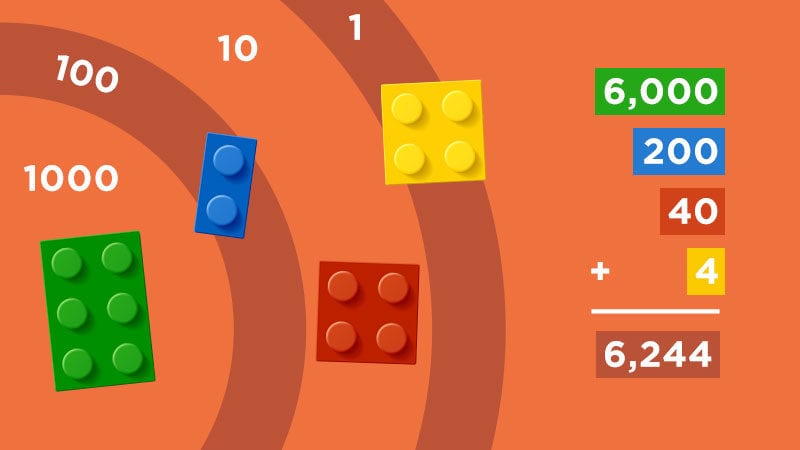Students toss LEGO bricks onto a bull’s-eye target (this can easily be made out of paper). Next, gather up bricks with different numbers of studs on top. The studs will represent numbers. Students use the studs on the Lego bricks and the place-value rings they land on to figure out their numbers. If two bricks land within the same ring, then kids count the studs on both bricks. We recommend one six- or eight-studded LEGO per toss.

## Play a LEGO dice game to practice math fluency.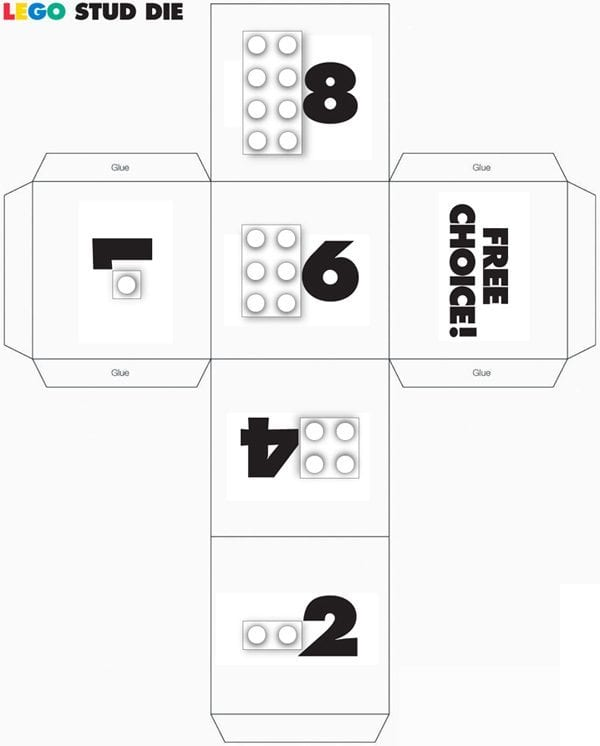For free printable LEGO dice, go here! Students can use a special LEGO die to explore greater than and less than, basic addition or subtraction, etc. They roll the die. Then, they take a LEGO brick that matches what the die lands on. Students could compare the studs on the LEGO bricks they collect as being greater than or less than. They could also create towers using a regular die. Each student gets 10 rolls. The student with the greatest tower wins!

For addition, students roll the LEGO die twice to create an addition or subtraction equation. Then, they solve it! They could also create multiplication equations. For older students working with two-digit numbers, have them roll the die four times to create two two-digit numbers. For example, if a student rolls a 4 and an 8, they create the number 48. Then, they roll a 2 and a 6 and make a 26. They must figure out 48 (x, +, or –) 26.

How do you use LEGO bricks to teach math in your classroom? Come and share in our WeAreTeachers HELPLINE group on Facebook.

Also, check out how to teach fractions with pool noodles.### Posted by Erin Bittman

Check out my blog eisforexplore.blogspot.com!

+